# Planar Motion

Narahari Narasapur

## Homework Statement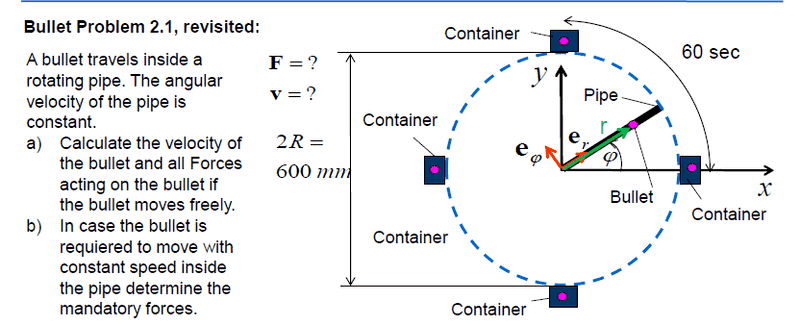## Homework Equations

https://wikimedia.org/api/rest_v1/media/math/render/svg/1a061d2667e5d9f69dc385e359e7260a3eb1deff
https://wikimedia.org/api/rest_v1/media/math/render/svg/ba88069934aa8abb2a2466f84121f385e6e8971f

## The Attempt at a Solution

I have solved equation for r(t) and i have arrived at: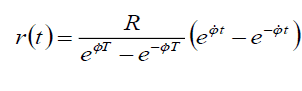Since the Bullet is moving freely the net forces acting on the bullet must be zero.
Radial component of the force is obviously zero since both Radial acceleration and centripetal acceleration are same and acting in opposite direction. In the tangential acceleration radial acceleration is zero since the pipe is rotating at constant speed.

But i am not understanding how coriolis force is becoming zero in tangential component.
Rather i am unable to find reason why coriolis force is zero!

Any insight into these concepts will be really helpful!
Thanks a lot

## Answers and Replies

Homework Helper
Gold Member
Since the Bullet is moving freely the net forces acting on the bullet must be zero.
I don't think that's what the question means. The bullet must be accelerating in order to stay within the rotating pipe, so the net force on it cannot be zero. Rather, I suggest it means that there is no radial force on the bullet.
Radial acceleration and centripetal acceleration are same and acting in opposite direction
No, they really are the same, i.e. two terms for the same thing, except that some people reserve the term centripetal for the case of constant radius. Note that "radial acceleration" means acceleration in the radial direction. It does not mean ##\ddot r##.
In the tangential acceleration radial acceleration is zero
I don't know what you mean by radial acceleration in the tangential acceleration.

Narahari Narasapur
I don't think that's what the question means. The bullet must be accelerating in order to stay within the rotating pipe, so the net force on it cannot be zero. Rather, I suggest it means that there is no radial force on the bullet.

No, they really are the same, i.e. two terms for the same thing, except that some people reserve the term centripetal for the case of constant radius. Note that "radial acceleration" means acceleration in the radial direction. It does not mean ##\ddot r##.

I don't know what you mean by radial acceleration in the tangential acceleration.
Thank you so much for your reply!
So do you suggest that, if the bullet is moving freely then it will not have radial acceleration. However, since the bullet must remain inside the pipe, net force cannot be zero. Hence Coriolis Acceleration exists?
I am looking at this acceleration equation here: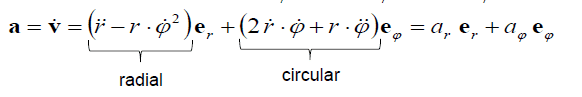And also about the velocity of the bullet, i have solved for r(t) (Position).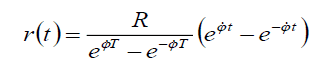Now, In case bullet is moving freely, the velocity of the bullet will have both tangential and radial component right?

Homework Helper
Gold Member
Thank you so much for your reply!
So do you suggest that, if the bullet is moving freely then it will not have radial acceleration. However, since the bullet must remain inside the pipe, net force cannot be zero. Hence Coriolis Acceleration exists?
I am looking at this acceleration equation here:
View attachment 102579

And also about the velocity of the bullet, i have solved for r(t) (Position).
View attachment 102580
Now, In case bullet is moving freely, the velocity of the bullet will have both tangential and radial component right?
You don't say how you obtained the equation for r(t). I would have thought it was by setting the radial acceleration to zero in the acceleration equation above. (There appears to be a typo in your r(t) equation. The exponents in the denominator should be ##\dot\phi T##, not ##\phi T##.). So I'm puzzled that you are questioning whether the radial acceleration is zero.

Not sure why you think Coriolis is relevant. As with centrifugal acceleration, that is a fictitious acceleration arising from the use of non-inertial frames. There is a tangential acceleration from the normal force from the pipe wall.

Narahari Narasapur
You don't say how you obtained the equation for r(t). I would have thought it was by setting the radial acceleration to zero in the acceleration equation above. (There appears to be a typo in your r(t) equation. The exponents in the denominator should be ##\dot\phi T##, not ##\phi T##.). So I'm puzzled that you are questioning whether the radial acceleration is zero.

Not sure why you think Coriolis is relevant. As with centrifugal acceleration, that is a fictitious acceleration arising from the use of non-inertial frames. There is a tangential acceleration from the normal force from the pipe wall.
You don't say how you obtained the equation for r(t). I would have thought it was by setting the radial acceleration to zero in the acceleration equation above. (There appears to be a typo in your r(t) equation. The exponents in the denominator should be ##\dot\phi T##, not ##\phi T##.). So I'm puzzled that you are questioning whether the radial acceleration is zero.

Not sure why you think Coriolis is relevant. As with centrifugal acceleration, that is a fictitious acceleration arising from the use of non-inertial frames. There is a tangential acceleration from the normal force from the pipe wall.

You are right! I calculated r(t) by setting radial acceleration to zero. Sorry for not mentioning that.. And I took screenshot of my r(t) equation! So
You don't say how you obtained the equation for r(t). I would have thought it was by setting the radial acceleration to zero in the acceleration equation above. (There appears to be a typo in your r(t) equation. The exponents in the denominator should be ##\dot\phi T##, not ##\phi T##.). So I'm puzzled that you are questioning whether the radial acceleration is zero.

Not sure why you think Coriolis is relevant. As with centrifugal acceleration, that is a fictitious acceleration arising from the use of non-inertial frames. There is a tangential acceleration from the normal force from the pipe wall.

Yes you are right! I got equation for r(t) by setting radial acceleration zero and also it is phi dot T. It's not visible in the screenshot. My bad :)

So the force acting on the bullet would be due to the tangential acceleration from the normal force from the pipe wall. That's the only force i have to mention. Right?
Can i say this the tangential acceleration is: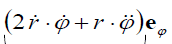Homework Helper
Gold Member
You are right! I calculated r(t) by setting radial acceleration to zero. Sorry for not mentioning that.. And I took screenshot of my r(t) equation! So

Yes you are right! I got equation for r(t) by setting radial acceleration zero and also it is phi dot T. It's not visible in the screenshot. My bad :)

So the force acting on the bullet would be due to the tangential acceleration from the normal force from the pipe wall. That's the only force i have to mention. Right?
Can i say this the tangential acceleration is:
View attachment 102592
Yes, but you can substitute in r(t), and you are told something about ##\dot\phi##.

Narahari Narasapur
That's clear now:)! Thank you haruspex for your suggestions!